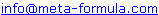# Metastock Formulas - B 1 Click here to go back to Metastock Formula Index

 IMPORTANT: These formulas aren't my complete collection. For my complete collection of instantly usable, profitable and powerful MetaStock formulas Click Here

Would You Like To Use MetaStock To Its Full Potential? Discover the Amazingly Simple Secret to Master Metastock Step-By-Step - click here

A | A 1 | B | B 1 | C | C 1 | D | D 1 | E | F | G | H | I | J | K | L | M | M 1 | N | O | P | R | S | S 1 | T | U | V | W | Z |

 Better Bollinger Bands Breaking out of Price Channels Breakout signals Bang For The Buck

## Better Bollinger Bands

In an article in Futures Magazine, October 1998, Dennis McNicholl describes the use of Bollinger Bands and provides a means of making them tighter when markets are trending. He calls them Better Bollinger Bands.

Here is the formula for MetaStock 6.5 or higher.

 pds:=Input("Periods",2,200,20); sd:=Input("Standard Deviations",.01,10,2); alpha:=2/(pds+1); mt:=alpha*C+(1-alpha)*(If(Cum(1)

(Go Top...)

## Breaking out of Price Channels

Breaking out of Price Channels, by Gerald Marisch, "Technical Analysis of Stocks & Commodities", January 1998, page 93.

"Here's a technique based upon Tushar Chande's variable-length moving average. The indicator is more responsive to market price movements than a conventional simple or exponential moving average, and can be used for position trading."

The following formula will match the authors slight modification to the variable moving average:

 VIDYA 21,5 Indicator Length:=Input("Length",1,200,21); Smooth:=Input("Smoothing",1,200,5); AbsCMO:=(Abs(CMO(C,Length)))/100; SC:=2/(Smooth+1); VIDYA:=If(Cum(1)<=(Length+1),C,(SC*AbsCMO*CLOSE)+(1-(SC*AbsCMO))*PREV); VIDYA

The following Expert highlights will show you when the price has undergone trend changes as discussed in the article. Enter each section as a separate highlight in an Expert Advisor. To do this, create a new Expert and select Highlights from the tab dialog. Then select New and name it Bull. Paste the Bull trend formula into the condition box within the editor and set the colour to green. Do this for the Bear and the Pause conditions as well, selecting the matching colours, Attach this Expert to your chart and if the conditions are met, the price bars will be displayed in the proper colours.

Green Bars (Bull trend)
 Length:=21; Smooth:=5; AbsCMO:=(Abs(CMO(C,Length)))/100; SC:=2/(Smooth+1); VIDYA:=If(Cum(1)<=(Length+1),C,(SC*AbsCMO*CLOSE)+(1-(SC*AbsCMO))*PREV); C>(Vidya*1.01)
Red Bars (Bear trend)
 Length:=21; Smooth:=5; AbsCMO:=(Abs(CMO(C,Length)))/100; SC:=2/(Smooth+1); VIDYA:=If(Cum(1)<=(Length+1),C,(SC*AbsCMO*CLOSE)+(1-(SC*AbsCMO))*PREV); C<(VIDYA*.99)
Yellow Bars (Pause or pending reversal of the trend)
 Length:=21; Smooth:=5; AbsCMO:=(Abs(CMO(C,Length)))/100; SC:=2/(Smooth+1); VIDYA:=If(Cum(1)<=(Length+1),C,(SC*AbsCMO*CLOSE)+(1-(SC*AbsCMO))*PREV); C>(VIDYA*.99) AND C<(VIDYA*1.01)

(Go Top...)

## Bang For The Buck

This indicator shows the possible dollar return (on a \$10,000 account) for a security on any given period. This is calculated by dividing a \$10,000 account by the closing price. This number is then multiplied by the average range of the security for the last 200 periods. The interpretation is such that the higher the value, the higher the profit potential.

((10000/C)* (Mov(ATR(1),200,S))/100)

(Go Top...)

## Breakout signals

{ Plots breakout long/short signals [email protected] With thanks to Roy Larsen for Init idea }

pds1:=Input("HHV (long) breakout periods",1,252,21);
pds2:=Input("LLV (short) breakout periods",1,252,10);
x:=Input("use Open=1 High=2 Low=3 Close=4 Volume=5 P=6",1,6,4);
delay:=Input("Entry and Exit delay",0,3,0);

x:=If(x=1,O,If(x=2,H,If(x=3,L,If(x=5,V,If(x=6,P,C)))));
In:=x>Ref(HHV(x,pds1),-1);
Out:=x<Ref(LLV(x,pds2),-1);

Init:=Cum(In+Out>-1)=1;
InInit:=Cum(In)=1;
In1:=Cum(Cum(In))=1;
Out1:=Cum(Cum(Out))=1;

If(display=1,Ref(Cum(Cum(In))=1,-delay),0);

Breakout signals
Signals a Buy Long on price breakout

Exploration filter

pds1:=21; {HHV (long) breakout periods}
pds2:=10; {LLV (short) breakout periods}
x:=4;   {use Open=1 High=2 Low=3 Close=4 Vol=5}

x:=If(x=1,O,If(x=2,H,If(x=3,L,If(x=5,V,C))));
In:=x>Ref(HHV(x,pds1),-1);
Out:=x<Ref(LLV(x,pds2),-1);

Init:=Cum(In+Out>-1)=1;
InInit:=Cum(In)=1;

Breakin signals
Signals a Sell Long on price collapse

Exploration filter

pds1:=21; {HHV (long) breakout periods}
pds2:=10; {LLV (short) breakout periods}
x:=4;   {use Open=1 High=2 Low=3 Close=4 Vol=5}

x:=If(x=1,O,If(x=2,H,If(x=3,L,If(x=5,V,C))));
In:=x>Ref(HHV(x,pds1),-1);
Out:=x<Ref(LLV(x,pds2),-1);

Init:=Cum(In+Out>-1)=1;
InInit:=Cum(In)=1;

SellLong

(Go Top...)

If you have Metastock formulas you would like to share,
Please email toWe look forward to hearing from you!